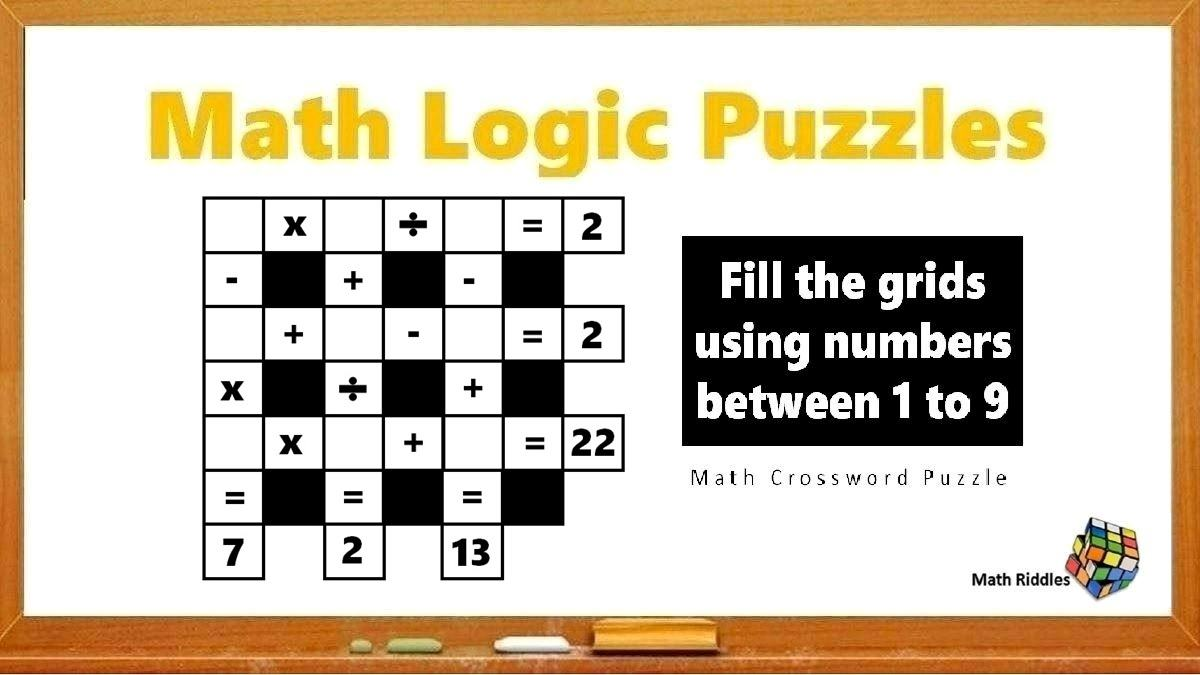# Math Riddles: Only 1% Can Solve This Math Crossword Puzzle, Difficulty Level Hard

Math Riddles Challenge: Using only numbers between 1 to 9, fill the empty puzzle boxes with the missing numbers in less than a 1 minute.Math Riddles: Only 1% Can Solve This Math Crossword Puzzle, Difficulty Level Hard

Math Riddles: Today, we have another math crossword puzzle to solve. In this math riddle series, you have to fill up the missing numbers in each of the empty boxes from numbers between 1 to 9 only such that they satisfy the equations in the question. Remember, no box can have repetition of numbers. Time for some brain exercise.

## Solution

Math Crossword Puzzle #1

Explanation:

Before we start solving, we have to keep in mind that calculations are done from left to right and top to bottom. Let us begin solving:

Taking up the Row C of the crossword puzzle, we figure the missing numbers between 1 to 9 that when multiplied and added gives us 22. So, we try all multiplication combinations while skipping repetitive products. While doing so, we get 7 x 3 + 1 however it does not satisfy our corresponding equations. Hence, we go with 7 x 2 + 8 = 22.

Moving on to column F, we figure out what numbers could possibly satisfy the equation to give us a 13. After trying a few possibilities, we get 9 – 4 + 8 = 13.

Next to Column E, we put 3 + 1 / 2 = 2. Next, we go to Row A, we already have a 3 and 9, so we can see a single candidate here that satisfies our equation. We put a 6 and see that 6 x 3 / 9 = 2.

Great job! We have figured out 90 percent of the math crossword. Upon scanning, we can see only one digit is left now which shall satisfy the equation in column D. So, we put the digit 5 and see that 6 – 5 x 7 = 7.

Horizontal Equations:

Row A => 6 x 3 / 9 = 2

Row B => 5 + 1 – 4 = 2

Row C => 7 x 2 + 8 = 22

Vertical Equations

Column D => 6 – 5 x 7 = 7

Column E => 3 + 1 / 2 = 2

Column F => 9 – 4 + 8 = 13

Now, our math crossword puzzle looks like this:

Math Crossword Solved!

## Tell us in comments: Did you solve this math crossword in less than 1 minute?

Check out more math puzzles!

Also Read: Sudoku Solver Tips & Tricks: How to Solve a Sudoku Puzzle Easy, Medium, Hard

Also Read: Math Riddles: Solve This Math Crossword Puzzle, Only with 1 to 9 Numbers

Also Try: Math Riddles: How Many Triangles Do You See In This Picture?

Also Read: Math Riddles: Can You Find the Missing Numbers in these Logic Puzzles?

Get the latest General Knowledge and Current Affairs from all over India and world for all competitive exams.
खेलें हर किस्म के रोमांच से भरपूर गेम्स सिर्फ़ जागरण प्ले पर